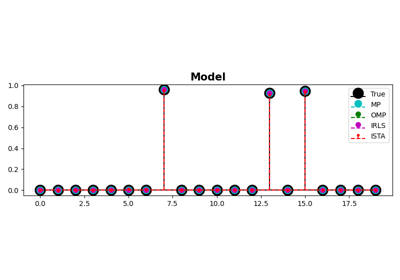# pylops.optimization.sparsity.OMP¶

pylops.optimization.sparsity.OMP(Op, data, niter_outer=10, niter_inner=40, sigma=0.0001, normalizecols=False, show=False)[source]

Orthogonal Matching Pursuit (OMP).

Solve an optimization problem with $$L0$$ regularization function given the operator Op and data y. The operator can be real or complex, and should ideally be either square $$N=M$$ or underdetermined $$N<M$$.

Parameters: Op : pylops.LinearOperator Operator to invert data : numpy.ndarray Data niter_outer : int, optional Number of iterations of outer loop niter_inner : int, optional Number of iterations of inner loop. By choosing niter_inner=0, the Matching Pursuit (MP) algorithm is implemented. sigma : list Maximum L2 norm of residual. When smaller stop iterations. normalizecols : list, optional Normalize columns (True) or not (False). Note that this can be expensive as it requires applying the forward operator $$n_{cols}$$ times to unit vectors (i.e., containing 1 at position j and zero otherwise); use only when the columns of the operator are expected to have highly varying norms. show : bool, optional Display iterations log xinv : numpy.ndarray Inverted model iiter : int Number of effective outer iterations cost : numpy.ndarray, optional History of cost function

ISTA
Iterative Shrinkage-Thresholding Algorithm (ISTA).
FISTA
Fast Iterative Shrinkage-Thresholding Algorithm (FISTA).
SPGL1
Spectral Projected-Gradient for L1 norm (SPGL1).
SplitBregman
Split Bregman for mixed L2-L1 norms.

Notes

Solves the following optimization problem for the operator $$\mathbf{Op}$$ and the data $$\mathbf{d}$$:

$||\mathbf{x}||_0 \quad subj. to \quad ||\mathbf{Op}\mathbf{x}-\mathbf{b}||_2^2 <= \sigma,$

using Orthogonal Matching Pursuit (OMP). This is a very simple iterative algorithm which applies the following step:

$\begin{split}\Lambda_k = \Lambda_{k-1} \cup \{ arg max_j |\mathbf{Op}_j^H \mathbf{r}_k| \} \\ \mathbf{x}_k = \{ arg min_{\mathbf{x}} ||\mathbf{Op}_{\Lambda_k} \mathbf{x} - \mathbf{b}||_2^2\end{split}$

Note that by choosing niter_inner=0 the basic Matching Pursuit (MP) algorithm is implemented instead. In other words, instead of solving an optimization at each iteration to find the best $$\mathbf{x}$$ for the currently selected basis functions, the vector $$\mathbf{x}$$ is just updated at the new basis function by taking directly the value from the inner product $$\mathbf{Op}_j^H \mathbf{r}_k$$.

In this case it is highly reccomended to provide a normalized basis function. If different basis have different norms, the solver is likely to diverge. Similar observations apply to OMP, even though mild unbalancing between the basis is generally properly handled.

## Examples using pylops.optimization.sparsity.OMP¶MP, OMP, ISTA and FISTA